# Fast Machine Learning Predictions

At BlaBlaCar, we want users to focus on sharing their rides and not spending time waiting for the search page to load. To achieve this goal the Trip Search team is working to create a fast and accurate search service. We’re also experimenting Machine Learning-powered search results in order to improve user matching. Our search service receives hundreds of queries per second and the response time is, on average, 60 milliseconds. We want to keep the response time as low as possible. However, keeping a low response time and applying Machine Learning predictions on thousands of trips for each of the hundreds of search queries per second is a challenging task.

Production-ready real-time prediction solutions do exist as SaaS. For instance Amazon real-time “responds to most (single) prediction requests within 100 milliseconds”. Google’s ML engine has a “maximum quota limits is 10K predictions per intervals of 100 seconds”. Besides, we do have an internal Machine Learning API, which responds at around 80 milliseconds for the prediction of a set of samples. However, those solutions end up being external to our production containers. That generates external requests adding a network time we can’t compress. Therefore prediction response time increases with the size of the samples due to the (de) serialization process.

We are moving to a Service Oriented Architecture in which the services are written in Java. Although R and Python’s ecosystems provide countless Machine Learning frameworks, that is not the case on Java’s. At BlaBlaCar, people working on Machine Learning modeling use R or Python, thus whatever our solution is, it must be able to read models from R and Python and perform predictions in Java. Although more and more languages run on the Java Virtual Machine (JVM), our solution must be written in Java to fully benefit from the Java ecosystem already in place.

Our goal is to have a library that can be quickly integrated into our services and that will perform predictions on the fly, thus no network requests required. The final result is a Java library I wrote and that we are experimenting with. It provides fast Machine Learning predictions as it aims to be used on the aforementioned scenario, e.g., real-time search experience, meeting points suggestions, etc. We aim to have a prediction response time of around 10 ms for a batch of samples, e.g., 1000 trips, meeting points, etc. The library is made to be agnostic of the prediction engine being used, currently supporting Extreme Gradient Boosting (XGBoost).

Next sections detail existing underlying libraries we evaluated, their pros, cons, a rather simple work-flow for each of them, and a benchmark suited to our use case.

### Libraries overview

R-Java

Pros

• The de-facto interface between Java and R
• Actively maintained, solid, more than 10 years of development
• Pre-processing could be real-time in R

Cons

• By using caret, the size of models exported from R may cause OutOfMemoryError
• No simple Python interaction, would imply on using a library such as reticulate

For each of the libraries’ workflow examples shown below, there will be a “R side” in which the model file is generated and a “Java side” in which the model is read and used to make the predictions. Of course, the model could be also have been equally generated using Python.

By using R-Java, the flow would be quite simple and yet powerful, i.e., fitting a model then saving its R’s object representation to a file, possibly with pre-processing capabilities given by caret, then reading it back into the Java service.

R’s side

`library(caret)`
`# Generate and export a modelmodel_fit <- glm(CLASS ~., data, family=binomial())saveRDS(model_fit, "model.R")`

Java’s side

`import org.rosuda.JRI.Rengine;private Rengine engine = Rengine.getMainEngine();`
`// Read model exported from the R sideString resource = getClass().getClassLoader().getResource("model.R").getFile();engine.assign("model_file", resource);`
`engine.eval("library(caret)");engine.eval("model_fit <- readRDS(model_file)");`
`// Perform the predictionREXP dataFrame = engine.eval("as.data.frame(predict(model_fit, newdata = data, type=\"prob\"))");`

JPMML

Pros

• Up-to-date implementation of the PMML spec
• Pre-processing out of the box
• Support models from R, Python, Tensorflow, …
• Integrates with Spark
• Actively maintained, used by AirBnB

Cons

• Basically a one-man-project, which creates a huge dependency on sole maintainer, although one can always get into the code and contribute

This library provides a nice out-of-the-box solution for the lack of interoperability between Machine Learning ecosystems on Python and R with Java. Models can be exported from single R functions such as glm to libaries as caret, Python’s scikit-learn, etc. Exporting models from R presents a work-flow similar to the R-Java’s one, for example

R’s side

`library(r2pmml)`
`# Generate and export a modelmodel_fit <- glm(CLASS ~., data, family=binomial())r2pmml(model_fit, "model.pmml"))`

Java’s side

`import org.dmg.pmml.PMML;import org.jpmml.evaluator.*;// Read model exported from the R sideInputStream resource = PMMLPredictor.class.getResourceAsStream("model.pmml");PMML pmml = org.jpmml.model.PMMLUtil.unmarshal(resource);ModelEvaluatorFactory modelEvaluatorFactory = ModelEvaluatorFactory.newInstance();evaluator = modelEvaluatorFactory.newModelEvaluator(pmml);`
`// Set the sample's dummy dataMap<FieldName, FieldValue> samples;`
`// Perform the predictionpredictions = evaluator.evaluate(samples);`

XGBoost Predictor

Pros

• Java implementation of XGBoost
Support models from R and Python
• Integrates with Spark

Cons

• Restricts to XGBoost models
• Per-sample prediction only
• No pre-processing

This library uniquely provides a XGBoost engine. Although it does not enable freedom of choice of engines as JPMML and R-Java, that is not a blocker, as our library architecture was conceived to wrap around multiple existing engines. Thus, if we are willing to add new engines, we could plug another library to that.

R side

`library(caret)library(xgboost)`
`model_fit <- # E.g., standard XGBoost train with caretbst <- xgboost:::xgb.Booster.check(model_fit\$finalModel, saveraw = FALSE)xgb.save(bst, fname = "model.xgb")`

Java side

`import biz.k11i.xgboost.Predictor;import biz.k11i.xgboost.util.FVec;`
`// Read model exported from the R sideInputStream resource = XGBoostPredictor.class.getResourceAsStream("model.xgb");`
`// Perform the (per-sample) predictionfor (int i = 0; i < numberOfSamples; i++) {    // Fill dummy dense matrix    double[] denseArray =      DoubleStream.generate(random::nextDouble).limit(numberOfColumns).toArray();    double[] denseArray = DoubleStream.generate(random::nextDouble).limit(numberOfFeatures).toArray();    featureVector = FVec.Transformer.fromArray(denseArray, false);    double[] prediction = predictor.predict(featureVector); }`

XGBoost4J

• Java implementation wrapping around XGBoost’s C++ implementation
• Actively maintained
• Support models from R and Python
• Integrates with Spark

Cons

• Restricts to XGBoost models
• No pre-processing
• Relies on native system library

Same remarks as for XGBoost Predictor. Furthermore, XGBoost4J’s native library dependency can be a real pain point for multi-archi usage. XGBoost4J’s work-flow is the same to XGBoost Predictor’s on the R side, though Java side changes a little as shown below

Java side

`import ml.dmlc.xgboost4j.java.Booster;import ml.dmlc.xgboost4j.java.DMatrix;import ml.dmlc.xgboost4j.java.XGBoost;`
`private Booster predictor;// Read model exported from the R sideInputStream resource = XGBoost4JPredictor.class.getResourceAsStream("model.xgb");predictor = XGBoost.loadModel(resource);float[] data = new float[numberOfFeatures * numberOfSamples];`
`// Fill dummy dense matrixDMatrix matrix = new DMatrix(data, numberOfRows, numberOfColumns);`
`// Perform the predictionfloat[][] predictions = predictor.predict(matrix);`

### Benchmark

I have explored 9 use cases (UC), which are the combinations of data frames with 100, 500, and 1000 rows (samples) and columns (features) using Logistic Regression (LR), Boosting Trees (GBM or XGBoost) to predict two-class categorical variables. Those UCs are what expect to be on the scale of our use cases at BlaBlaCar, e.g., up to a 1000 meeting points to rank containing up to a 1000 features.

Results below are presented with 95 CI, error bars are Standard Error of the Mean (SEM) for 500 repeats. For LR, GBM and XGBoost we make predictions on those 9 use cases. Table below represents the aforementioned use cases.

`          ╔═══════════════════════════════════════════╗          ║        Number of columns (features)       ║          ╠═══════════════╦══════╦══════╦══════╦══════╣          ║               ║      ║ 100  ║ 500  ║ 1000 ║           ║   Number of   ║══════╬══════╬══════╬══════╣          ║     rows      ║ 100  ║ UC 1 ║ UC 4 ║ UC 7 ║          ║   (samples)   ║ 500  ║ UC 2 ║ UC 5 ║ UC 8 ║          ║               ║ 1000 ║ UC 3 ║ UC 6 ║ UC 9 ║          ╚═══════════════╩════════════════════╩══════╝`

The sole metric I have explored is the prediction time. It means that the time to build the sample’s data was not evaluated, as it may vary enormously given one’s requirements. For instance, composing single features, e.g., duration of a trip related to its deviation for the group may be fast to compute, but if there are hundreds of derivate features to be generated before the prediction, this pre-processing will likely take much more time.

Therefore, to illustrate the time calculation in one of the Java examples above, the prediction time is calculated as following

`// Fill dummy dense matrixDMatrix matrix = new DMatrix(data, numberOfRows, numberOfColumns);`
`// Perform the prediction`
`Instant start = Instant.now();float[][] predictions = predictor.predict(matrix);Instant end = Instant.now();`
`long repeatDuration = Duration.between(start, end).toMillis();`

#### Logistic Regression’s benchmark

We have started our exploration of Machine Learning modeling at BlaBlaCar by using Logistic Regression models. Thus, I wanted to see how each of libraries behave on each of the UCs. For this comparison XGBoost has been applied with `objective = "binary:logistic”`and`nrounds = 1`.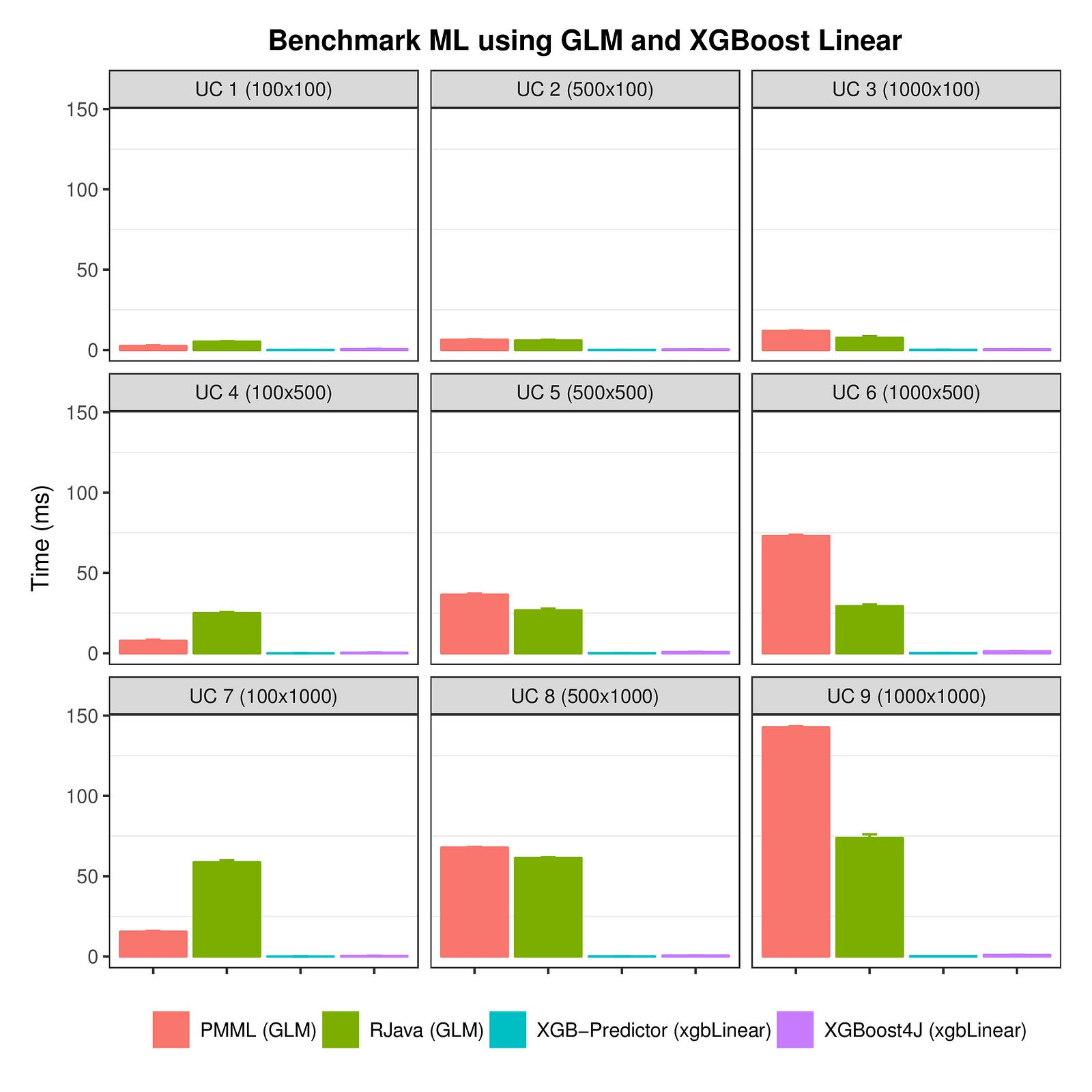Prediction time per library using logistic models faceted by UC

The chart above shows that R-Java and PMML are less performant on “harder” use cases, i.e., those with more samples and features. Below we have the same chart, but faceted by library this time, it will show clearly how each of them behave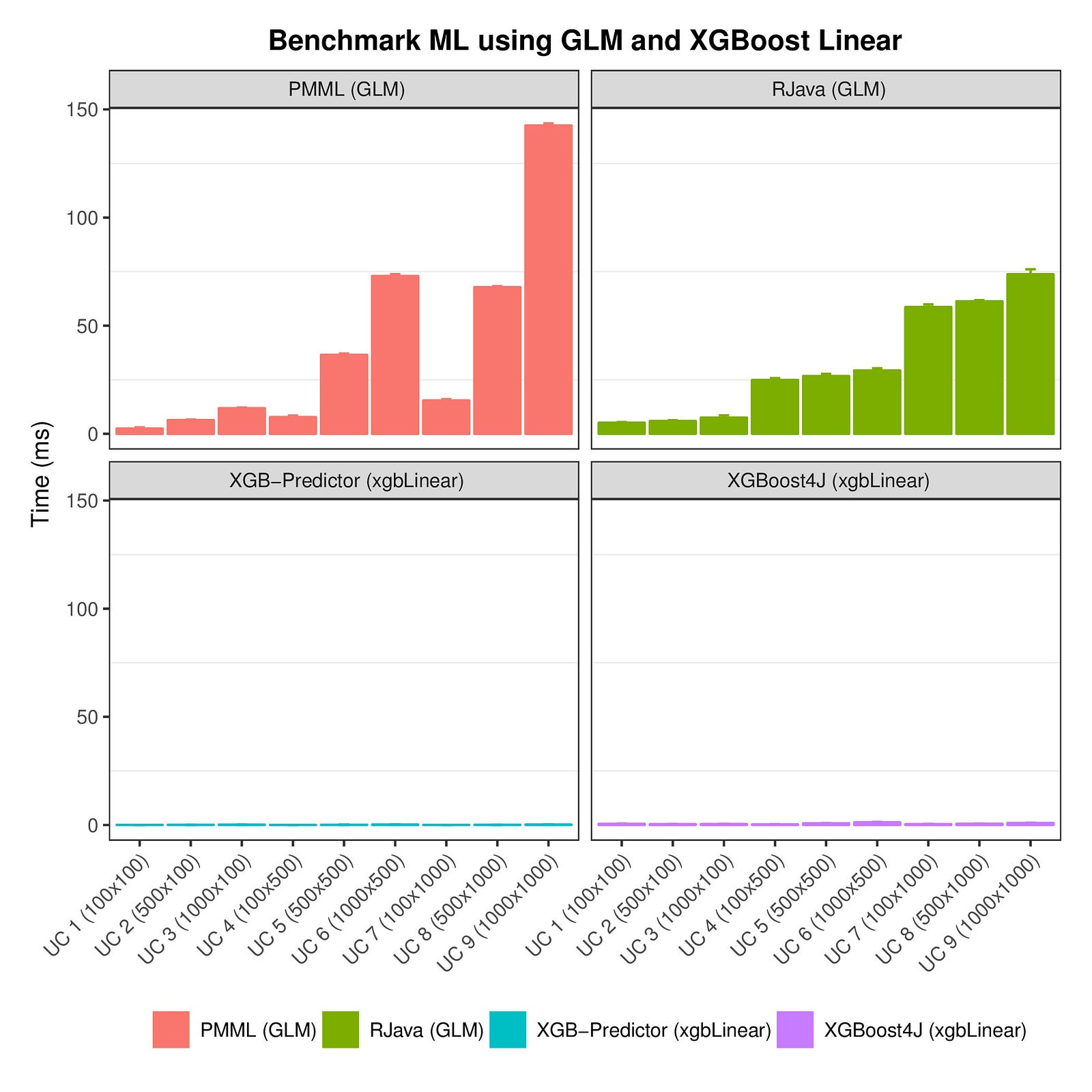Prediction time per library using logistic models faceted by library

As illustrated above, the prediction time increases when more data needs to be predicted on PMML and R-Java for the tested UCs. That does not mean that XGBoost libraries do not follow the same rule, but it might be that the UCs explored here are not as large in terms of features and samples as needed to see this trend also for them.

If our goal was to predict up to a hundred samples with GLM only, i.e., cases covered in UC1, UC2 and UC3 (zoom below), any of the libraries would be OK, around 12 milliseconds is fair enough.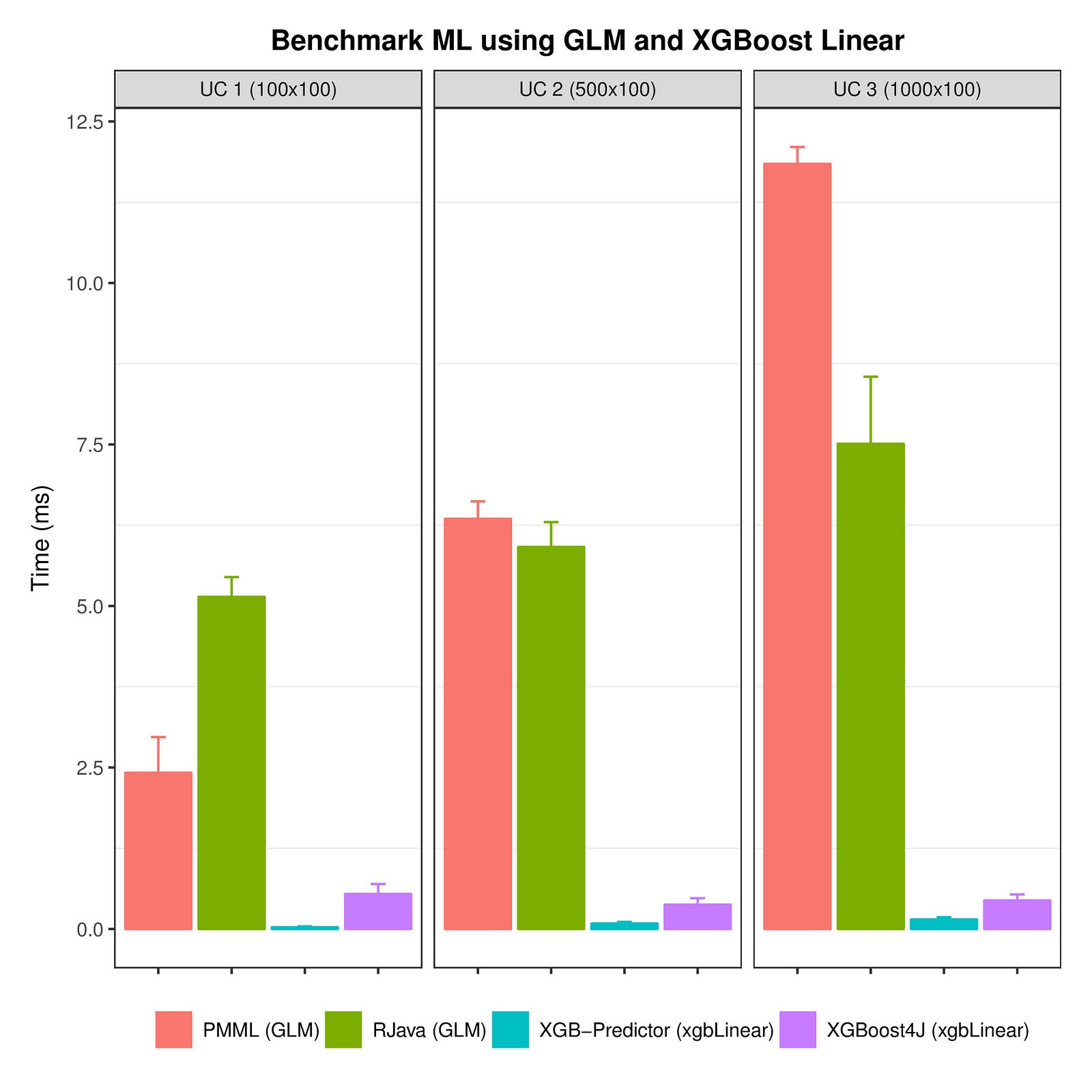Zooming into the first three UCs

To wrap-up the linear benchmark we have XGBoost libraries predicting way faster than RJava and PMML. Besides,

• PMML very sensible to the increase of features and samples
• RJava very sensible to the increase of samples
• XGBoost4J and XGBoost Predictor stable times regardless use case

#### Boosting Tress Benchmark

Boosting trees do not need introduction, they provide black-box like powerful prediction. We start out below with the same kind of chart from the linear benchmark to have an insight on the per-UC performances. For this comparison XGBoost has been applied with `objective = "binary:logistic”`and`nrounds = 150`.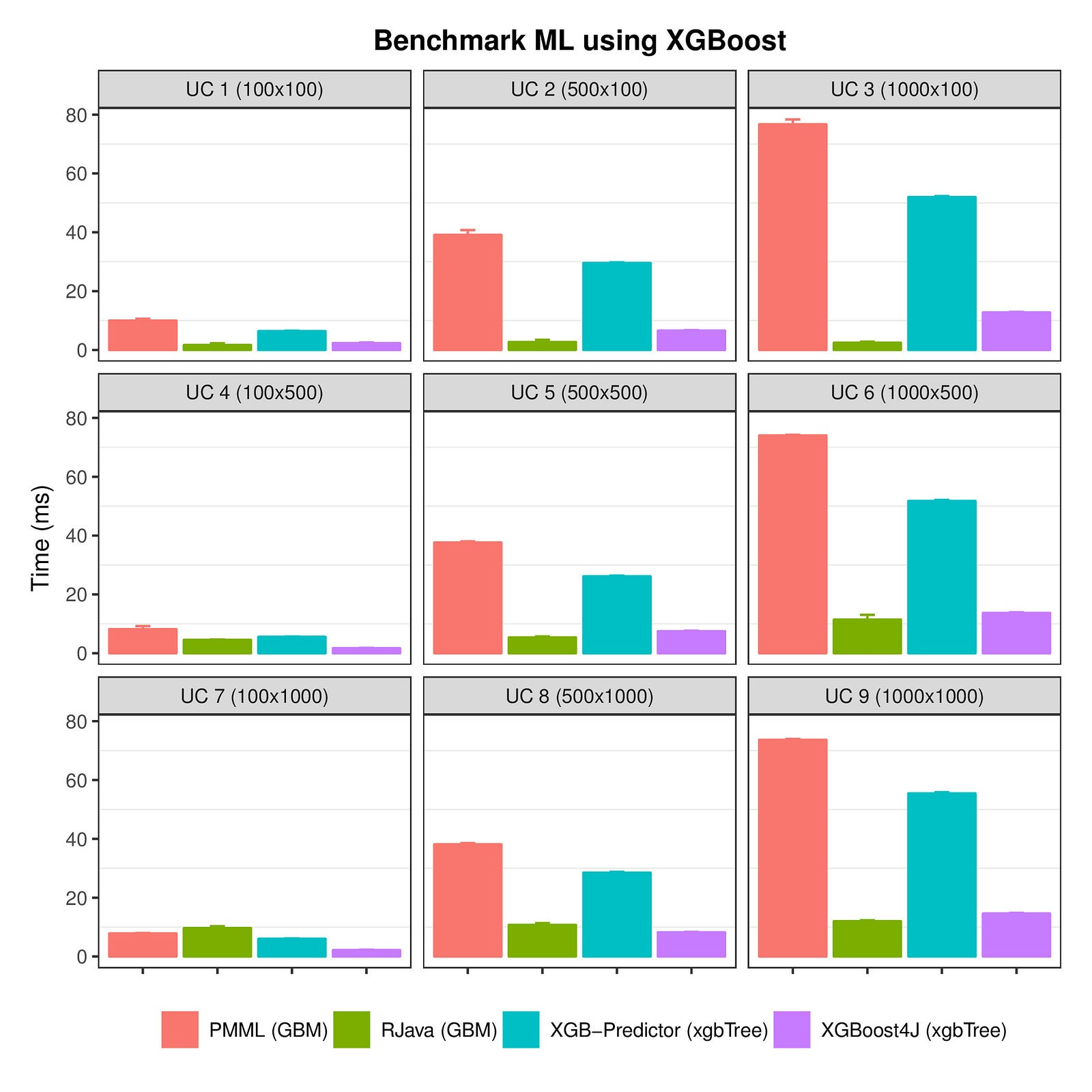Prediction time per library using boosting models faceted by UC

This time XGBoost Predictor and PMML have their prediction time increased with the number of samples, figure below better illustrates that by faceting per library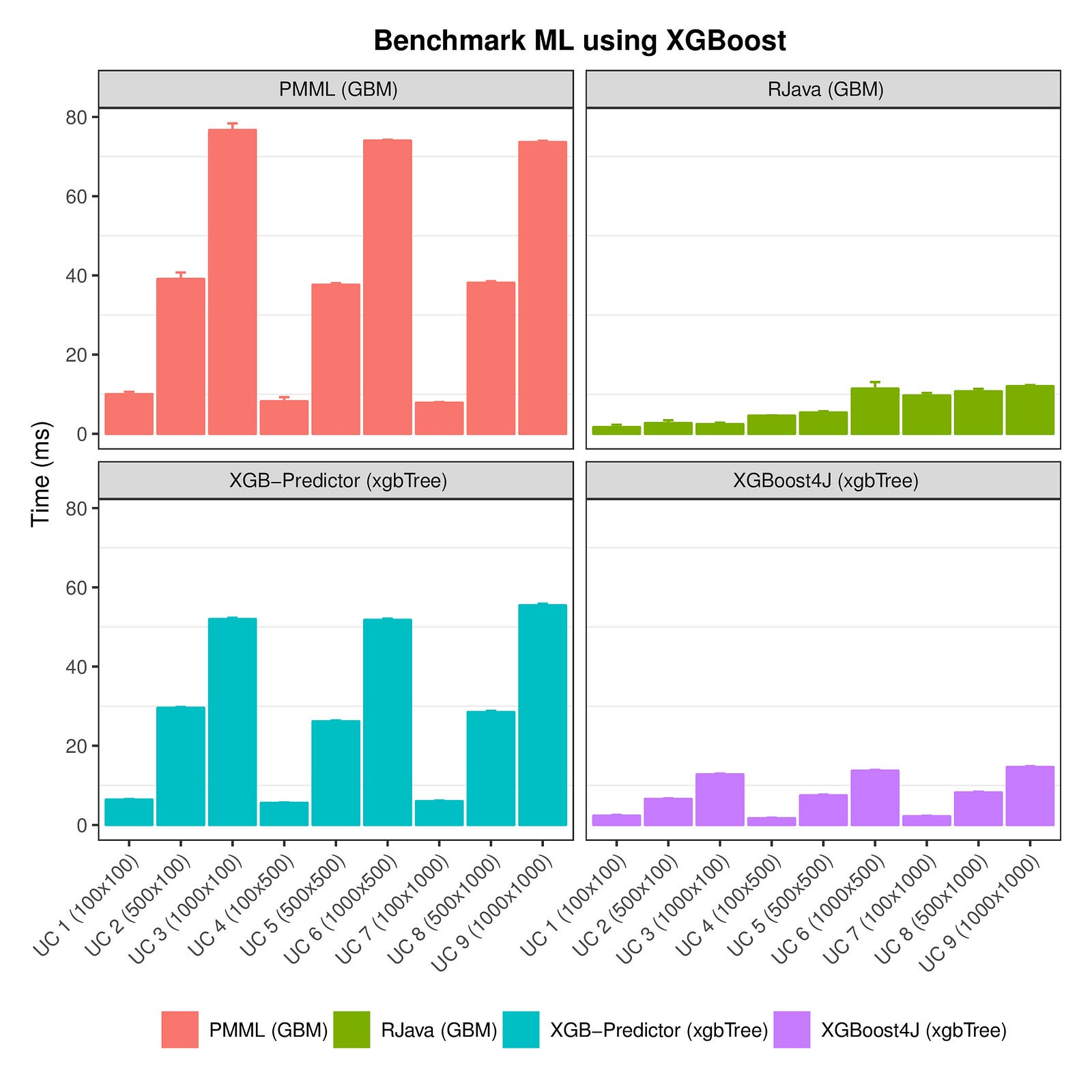Prediction time per library using boosting models faceted by library

The results show that, for boosting trees, XGBoost Predictor and PMML had way slower prediction times by increasing the number of samples.

• PMML and XGBoost Predictor very sensible to the increase of samples. 100 to 500 implied ~ 4.5 increase on prediction time. 500 to 1000 implied ~ 1.9 increase on prediction time
• XGBoost4J and RJava stable times regardless the tested use cases
• At least for the UCs evaluated here, XGBoost Predictor does not perform as it claims on its repository “[XGBoost Predictor] is about 6,000 to 10,000 times faster than XGBoost4J on prediction tasks”. It actually performed worse than XGBoost4J. Similar results have been mentioned in this discussion

### Our library design

As new projects using Machine Learning are started, people may not want to start with more complex boosting models. Thus GLM needs to be supported by the library as well, so its aforementioned results must be considered here.

XGBoost4J has been selected as the first underlying library of our internal library given prediction speed is our main goal.

Although the library is not yet open as we are integrating and testing it internally, it is used as follows

`// Read model exported from the R side and create predictorInputStream modelResource = getClass().getClassLoader().getResourceAsStream("model.XGB");`
`MachineLearningOperationBuilder predictor = MachineLearning.newXGB(modelResource);`
`// Tuple's key help to glue with the business object later and have a list of featuresTuple featTuple1 = new Tuple("trip1", Lists.newArrayList(0f, 1f, 3f, 5f));Tuple featTuple2 = new Tuple("trip2", Lists.newArrayList(0f, 2f, 5f, 5f));Tuple featTuple3 = new Tuple("trip3", Lists.newArrayList(0f, 3f, 7f, 5f));`
`// Create data frame from the given tuplesFeatureDataFrame featureDataFrame = new FeatureDataFrame(Lists.newArrayList(featTuple1, featTuple2, featTuple3));`
`// Perform predictionProbabilityDataFrame probDataFrame = predictor.with(featureDataFrame)          .predict();`

Considering a 3-class problem the `probDataFrame` would contain something in the lines of the extract below, i.e., one probability per class. Then a comparator can be created in order to sort them by their probability of a certain class.

`Tuple(id=trip1, features=[0.033174984, 0.0935384, 0.8732866])Tuple(id=trip2, features=[0.05029368, 0.055675365, 0.894031])Tuple(id=trip3, features=[0.12031816, 0.27852917, 0.60115266])`

The library currently supports post processing by the means of a lambda passed to the method `score` which, under the hood, applies `predict().score(lambda)` i.e., chains the given lambda as exemplified below.

`PostProcessor<ProbabilityDataFrame> multiplier = (features) -> new ProbabilityDataFrame(        features.getTuples()                .stream()                .map(row -> new Tuple(row.getId(),                        Lists.newArrayList((float) row                                .getFeatures()                                .stream()                                .mapToDouble(aFloat -> aFloat)                                .sum()))                ).collect(Collectors.toList()));ProbabilityDataFrame probDataFrame = machineLearningOperation.with(featureDataFrame)        .score(multiplier);`

The example above sums each of the per-class probabilities. Although rather contrived, it illustrates how post processing can be implemented by passing functions defined by the client, giving complete flexibility to the user of the library.

`// Illustrative result of the function aboveTuple(id=trip1, features=)Tuple(id=trip2, features=)Tuple(id=trip3, features=)`

### Some results

Results below show the elapsed time for each of the two main steps on a recommendation in production at BlaBlaCar, prediction (“predict”) and feature crunching (“features”), e.g., matching a category to corresponding ordinal used on the training phase, attributing default to null-valued attributes from the input query, etc. To give a bit more of context, this model predicts which are the meeting points that should be suggested for a driving while publishing a ride. The model has 7 features.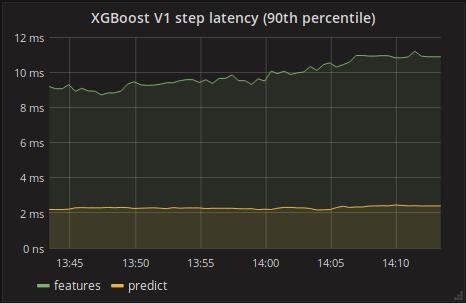Elapsed time for feature crunching on yellow and prediction on green.

### Conclusions

At BlaBlaCar we are at the beginning of a transformation in which more and more services will apply Machine Learning to improve the quality of the response it gives to the users. Given the widespread of R and Python on this field, it is not always easy to choose the best approach on how to create an interaction between them and Java services. Besides, if prediction speed is an important aspect, that becomes a very challenging task. The goal of this article was to explore the possibilities considering prediction speed as the main goal.

Nonetheless, depending on different needs, e.g., a less constrained response time, other choices are more suitable. For instance if hundreds of milliseconds of prediction time is not a problem, I recommend using PMML. It gives an ultra-wide choice of libraries it can read exported models from. If those training the models solely use R, I’d recommend R-Java or PMML. The latter because embedding R code into the Java code base may quickly become messy, unless it strict encapsulation design separates them as much as possible.

Although the benchmark results seem clear enough, one last point intrigued me and I have no explanation for it. When comparing R-Java’s GBM and GLM, the former has faster response time. It is not a R-Java related behavior. By launching the same comparison inside R’s environment `replicate(500, system.time(predict(model_fit, test)))`, where `model_fit` is the same model given to R-Java’s benchmark and `test` being the same test we have the same results indicating that GLM is slower on the prediction. I have not explored further, though.

There are some clear next steps:

• Create a pre-processing pipeline to be able to deal with the simplest tasks, such as one-hot encoding, etc
• Improve model integration, e.g., easier synchronization of feature order between people who train the models and the ones implementing it into the service. It gets pretty hard to keep things synchronized when when you have hundreds of features